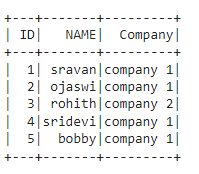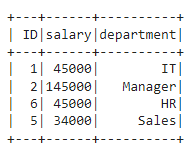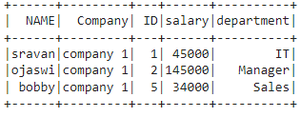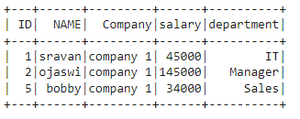# How to avoid duplicate columns after join in PySpark ?

• Last Updated : 19 Dec, 2021

In this article, we will discuss how to avoid duplicate columns in DataFrame after join in PySpark using Python.

## Python3

 `# importing module``import` `pyspark`` ` `# importing sparksession from pyspark.sql module``from` `pyspark.sql ``import` `SparkSession`` ` `# creating sparksession and giving an app name``spark ``=` `SparkSession.builder.appName(``'sparkdf'``).getOrCreate()`` ` `# list  of employee data``data ``=` `[[``"1"``, ``"sravan"``, ``"company 1"``],``        ``[``"2"``, ``"ojaswi"``, ``"company 1"``],``        ``[``"3"``, ``"rohith"``, ``"company 2"``],``        ``[``"4"``, ``"sridevi"``, ``"company 1"``],``        ``[``"5"``, ``"bobby"``, ``"company 1"``]]`` ` `# specify column names``columns ``=` `[``'ID'``, ``'NAME'``, ``'Company'``]`` ` `# creating a dataframe from the lists of data``dataframe ``=` `spark.createDataFrame(data, columns)`` ` `dataframe.show()`

Output:## Python3

 `# list  of employee data``data1 ``=` `[[``"1"``, ``"45000"``, ``"IT"``],``         ``[``"2"``, ``"145000"``, ``"Manager"``],``         ``[``"6"``, ``"45000"``, ``"HR"``],``         ``[``"5"``, ``"34000"``, ``"Sales"``]]`` ` `# specify column names``columns ``=` `[``'ID'``, ``'salary'``, ``'department'``]`` ` `# creating a dataframe from the lists of data``dataframe1 ``=` `spark.createDataFrame(data1, columns)`` ` `dataframe1.show()`

Output:## Method 1: Using drop() function

We can join the dataframes using joins like inner join and after this join, we can use the drop method to remove one duplicate column.

Syntax: dataframe.join(dataframe1,dataframe.column_name == dataframe1.column_name,”inner”).drop(dataframe.column_name)

where,

• dataframe is the first dataframe
• dataframe1 is the second dataframe
• inner specifies inner join
• drop() will delete the common column and delete first dataframe column

## Python3

 `# inner join on two dataframes``# and remove duplicate column``dataframe.join(dataframe1,``               ``dataframe.``ID` `=``=` `dataframe1.``ID``,``               ``"inner"``).drop(dataframe.``ID``).show()`

Output:## Method 2: Using join()

Here we are simply using join to join two dataframes and then drop duplicate columns.

Syntax: dataframe.join(dataframe1, [‘column_name’]).show()

where,

• dataframe is the first dataframe
• dataframe1 is the second dataframe
• column_name is the common column exists in two dataframes

## Python3

 `# join on two dataframes``# and remove duplicate column``dataframe.join(dataframe1, [``'ID'``]).show()`

Output:My Personal Notes arrow_drop_up